JMSLTM Numerical Library 7.2.0
com.imsl.math

## Class SparseLP

• All Implemented Interfaces:
Serializable, Cloneable

```public class SparseLP
extends Object
implements Serializable, Cloneable```
Solves a sparse linear programming problem by an infeasible primal-dual interior-point method.

Class `SparseLP` uses an infeasible primal-dual interior-point method to solve linear programming problems, i.e., problems of the form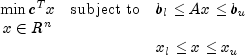where c is the objective coefficient vector, A is the coefficient matrix, and the vectors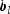,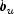,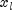, and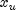are the lower and upper bounds on the constraints and the variables, respectively.

Internally, `SparseLP` transforms the problem given by the user into a simpler form that is computationally more tractable. After redefining the notation, the new form reads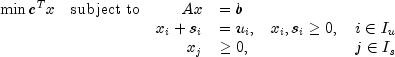Here,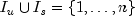is a partition of the index set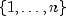into upper bounded and standard variables.

In order to simplify the description it is assumed in the following that the problem above contains only variables with upper bounds, i.e. is of the form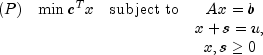The corresponding dual problem is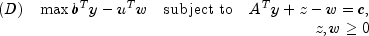The Karush-Kuhn-Tucker (KKT) optimality conditions for (P) and (D) are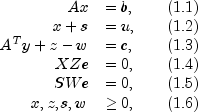where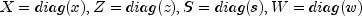are diagonal matrices and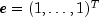is a vector of ones.

Class `SparseLP`, like all infeasible interior-point methods, generates a sequence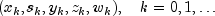of iterates that satisfy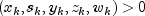for all k, but are in general not feasible, i.e. the linear constraints (1.1)-(1.3) are only satisfied in the limiting case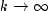.

The barrier parameter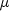, defined by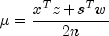measures how good the complementarity conditions (1.4), (1.5) are satisfied.

Mehrotra's predictor-corrector algorithm is a variant of Newton's method applied to the KKT conditions (1.1)-(1.5). Class `SparseLP` uses a modified version of this algorithm to compute the iterates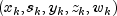. In every step of the algorithm, the search direction vector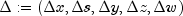is decomposed into two parts,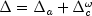, where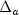and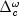denote the affine-scaling and the weighted centering components, respectively. Here,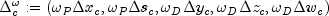where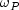and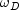denote the primal and dual corrector weights, respectively.

The vectorsand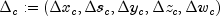are determined by solving the linear system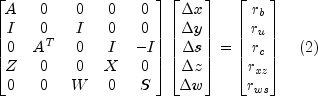for two different right-hand sides.

For, the right-hand side is defined as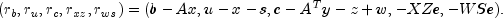Here,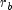and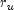are the violations of the primal constraints and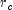defines the violations of the dual constraints.

The resulting directionis the pure Newton step applied to the system (1.1)-(1.5).

In order to obtain the corrector direction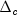, the maximum stepsizes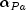in the primal and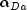in the dual space preserving nonnegativity of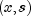and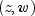respectively, are determined, and the predicted complementarity gap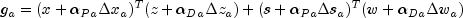is computed. It is then used to determine the barrier parameter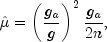where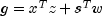denotes the current complementarity gap.

The directionis then computed by choosing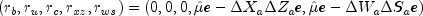as the right-hand side in the linear system (2).

Class `SparseLP` now uses a line search to find the optimal weight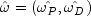that maximizes the stepsizes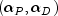in the primal and dual directions of, respectively.

A new iterate is then computed using a step reduction factor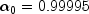: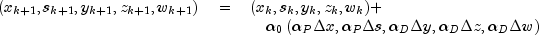In addition to the weighted Mehrotra predictor-corrector, `SparseLP` also uses multiple centrality correctors to enlarge the primal-dual stepsizes per iteration step and to reduce the overall number of iterations required to solve an LP problem. The maximum number of centrality corrections depends on the ratio of the factorization and solve efforts for system (2) and is therefore problem dependent. For a detailed description of multiple centrality correctors, refer to Gondzio (1994).

The linear system (2) can be reduced to more compact forms, the augmented system (AS)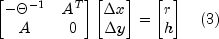or further by elimination of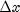to the normal equations (NE) system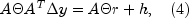where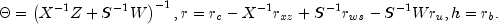The matrix on the left-hand side of (3), which is symmetric indefinite, can be transformed into a symmetric quasidefinite matrix by regularization. Since these types of matrices allow for a Cholesky-like factorization, the resulting linear system can be solved easily for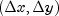by triangular substitutions. For more information on the regularization technique, see Altman and Gondzio (1998). For the NE system, matrix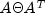is positive definite, and therefore a sparse Cholesky algorithm can be used to factorand solve the system for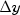by triangular substitutions with the Cholesky factor L.

In class `SparseLP`, both approaches are implemented. The AS approach is chosen if A contains dense columns, if there are a considerable number of columns in A that are much denser than the remaining ones, or if there are many more rows than columns in the structural part of A. Otherwise, the NE approach is selected.

Class `SparseLP` stops with optimal termination status if the current iterate satisfies the following three conditions: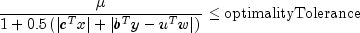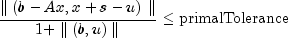and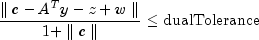where `primalTolerance`, `dualTolerance` and `optimalityTolerance` are primal infeasibility, dual infeasibility and optimality tolerances, respectively. The default value is 1.0e-10 for `optimalityTolerance` and 1.0e-8 for the other two tolerances.

Class `SparseLP` is based on the code HOPDM developed by Jacek Gondzio et al., see the HOPDM User's Guide (1995).

Example 1, Example 2, Serialized Form
• ### Nested Class Summary

Nested Classes
Modifier and Type Class and Description
`static class ` `SparseLP.CholeskyFactorizationAccuracyException`
The Cholesky factorization failed because of accuracy problems.
`static class ` `SparseLP.DiagonalWeightMatrixException`
A diagonal element of the diagonal weight matrix is too small.
`static class ` `SparseLP.DualInfeasibleException`
The dual problem is infeasible.
`static class ` `SparseLP.IllegalBoundsException`
The lower bound is greater than the upper bound.
`static class ` `SparseLP.IncorrectlyActiveException`
One or more LP variables are falsely characterized by the internal presolver.
`static class ` `SparseLP.IncorrectlyEliminatedException`
One or more LP variables are falsely characterized by the internal presolver.
`static class ` `SparseLP.InitialSolutionInfeasibleException`
The initial solution for the one-row linear program is infeasible.
`static class ` `SparseLP.PrimalInfeasibleException`
The primal problem is infeasible.
`static class ` `SparseLP.PrimalUnboundedException`
The primal problem is unbounded.
`static class ` `SparseLP.ProblemUnboundedException`
The problem is unbounded.
`static class ` `SparseLP.TooManyIterationsException`
The maximum number of iterations has been exceeded.
`static class ` `SparseLP.ZeroColumnException`
A column of the constraint matrix has no entries.
`static class ` `SparseLP.ZeroRowException`
A row of the constraint matrix has no entries.
• ### Constructor Summary

Constructors
Constructor and Description
```SparseLP(int[] colPtr, int[] rowInd, double[] values, double[] b, double[] c)```
Constructs a `SparseLP` object using Compressed Sparse Column (CSC), or Harwell-Boeing format.
`SparseLP(MPSReader mps)`
Constructs a `SparseLP` object using an `MPSReader` object.
```SparseLP(SparseMatrix a, double[] b, double[] c)```
Constructs a `SparseLP` object.
• ### Method Summary

Methods
Modifier and Type Method and Description
`double` `getConstant()`
Returns the value of the constant term in the objective function.
`int[]` `getConstraintType()`
Returns the types of general constraints in the matrix A.
`double` `getDualInfeasibility()`
Returns the dual infeasibility of the solution.
`double` `getDualInfeasibilityTolerance()`
Returns the dual infeasibility tolerance.
`double[]` `getDualSolution()`
Returns the dual solution.
`int` `getIterationCount()`
Returns the number of iterations used by the primal-dual solver.
`double` `getLargestCPRatio()`
Returns the ratio of the largest complementarity product to the average.
`double[]` `getLowerBound()`
Returns the lower bound on the variables.
`int` `getMaxIterations()`
Returns the maximum number of iterations allowed for the primal-dual solver.
`double` `getOptimalValue()`
Returns the optimal value of the objective function.
`int` `getPreordering()`
Returns the variant of the Minimum Degree Ordering (MDO) algorithm used in the preordering of the normal equations or augmented system matrix.
`int` `getPresolve()`
Returns the presolve option.
`double` `getPrimalInfeasibility()`
Returns the primal infeasibility of the solution.
`double` `getPrimalInfeasibilityTolerance()`
Returns the primal infeasibility tolerance.
`int` `getPrintLevel()`
Returns the print level.
`double` `getRelativeOptimalityTolerance()`
Returns the relative optimality tolerance.
`double` `getSmallestCPRatio()`
Returns the ratio of the smallest complementarity product to the average.
`double[]` `getSolution()`
Returns the solution x of the linear programming problem.
`int` `getTerminationStatus()`
Returns the termination status for the problem.
`double[]` `getUpperBound()`
Returns the upper bound on the variables.
`double[]` `getUpperLimit()`
Returns the upper limit of the constraints that have both a lower and an upper bound.
`double` `getViolation()`
Returns the violation of the variable bounds.
`void` `setConstant(double c0)`
Sets the value of the constant term in the objective function.
`void` `setConstraintType(int[] constraintType)`
Sets the types of general constraints in the matrix A.
`void` `setDualInfeasibilityTolerance(double dualTolerance)`
Sets the dual infeasibility tolerance.
`void` `setLowerBound(double[] lowerBound)`
Sets the lower bound on the variables.
`void` `setMaxIterations(int maxIterations)`
Sets the maximum number of iterations allowed for the primal-dual solver.
`void` `setPreordering(int preorder)`
Sets the variant of the Minimum Degree Ordering (MDO) algorithm used in the preordering of the normal equations or augmented system matrix.
`void` `setPresolve(int presolve)`
Sets the presolve option.
`void` `setPrimalInfeasibilityTolerance(double primalTolerance)`
Sets the primal infeasibility tolerance.
`void` `setPrintLevel(int printLevel)`
Sets the print level.
`void` `setRelativeOptimalityTolerance(double optimalityTolerance)`
Sets the relative optimality tolerance.
`void` `setUpperBound(double[] upperBound)`
Sets the upper bound on the variables.
`void` `setUpperLimit(double[] bu)`
Sets the upper limit of the constraints that have both a lower and an upper bound.
`void` `solve()`
Solves the sparse linear programming problem by an infeasible primal-dual interior-point method.
• ### Methods inherited from class java.lang.Object

`clone, equals, finalize, getClass, hashCode, notify, notifyAll, toString, wait, wait, wait`
• ### Constructor Detail

• #### SparseLP

```public SparseLP(int[] colPtr,
int[] rowInd,
double[] values,
double[] b,
double[] c)```
Constructs a `SparseLP` object using Compressed Sparse Column (CSC), or Harwell-Boeing format. See Compressed Sparse Column (CSC) Format, Chapter 1.
Parameters:
`colPtr` - an `int` array containing the location in `values` in which to place the first nonzero value of each succeeding column of the constraint matrix A. `colPtr`, `rowInd` and `values` specify the location and value of each nonzero coefficient in the constraint matrix A in CSC format.
`rowInd` - an `int` array containing a list of the row indices of each column of the constraint matrix A. `colPtr`, `rowInd` and `values` specify the location and value of each nonzero coefficient in the constraint matrix A in CSC format.
`values` - a `double` array containing the value of each nonzero coefficient in the constraint matrix A. `colPtr`, `rowInd` and `values` specify the location and value of each nonzero coefficient in the constraint matrix A in CSC format.
`b` - a `double` array of length m, number of constraints, containing the right-hand side of the constraints; if there are limits on both sides of the constraints, then `b` contains the lower limit of the constraints
`c` - a `double` array of length n, the number of variables, containing the coefficients of the objective function
• #### SparseLP

`public SparseLP(MPSReader mps)`
Constructs a `SparseLP` object using an `MPSReader` object.
Parameters:
`mps` - an `MPSReader` object specifying the Linear Programming problem
• #### SparseLP

```public SparseLP(SparseMatrix a,
double[] b,
double[] c)```
Constructs a `SparseLP` object.
Parameters:
`a` - a `SparseMatrix` object containing the location and value of each nonzero coefficient in the constraint matrix A. If there is no constraint matrix, set `a = null`.
`b` - a `double` array of length m, the number of constraints, containing the right-hand side of the constraints. If there are limits on both sides of the constraints, then `b` contains the lower limit of the constraints.
`c` - a `double` array of length n, the number of variables, containing the coefficients of the objective function
• ### Method Detail

• #### getConstant

`public double getConstant()`
Returns the value of the constant term in the objective function.
Returns:
a `double` scalar containing the value of the constant term in the objective function
• #### getConstraintType

`public int[] getConstraintType()`
Returns the types of general constraints in the matrix A. See `setConstraintType`.
Returns:
an `int` array containing the types of general constraints in the matrix A
• #### getDualInfeasibility

`public double getDualInfeasibility()`
Returns the dual infeasibility of the solution.
Returns:
a `double` scalar containing the dual infeasibility of the solution,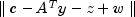• #### getDualInfeasibilityTolerance

`public double getDualInfeasibilityTolerance()`
Returns the dual infeasibility tolerance.
Returns:
a `double` scalar containing the dual infeasibility tolerance
• #### getDualSolution

`public double[] getDualSolution()`
Returns the dual solution.
Returns:
a `double` array containing the dual solution
• #### getIterationCount

`public int getIterationCount()`
Returns the number of iterations used by the primal-dual solver.
Returns:
an `int` scalar containing the number of iterations used by the primal-dual solver
• #### getLargestCPRatio

`public double getLargestCPRatio()`
Returns the ratio of the largest complementarity product to the average.
Returns:
a `double` scalar containing the ratio of the largest complementarity product to the average
• #### getLowerBound

`public double[] getLowerBound()`
Returns the lower bound on the variables.
Returns:
a `double` array containing the lower bound on the variables
• #### getMaxIterations

`public int getMaxIterations()`
Returns the maximum number of iterations allowed for the primal-dual solver.
Returns:
an `int` scalar containing the maximum number of iterations allowed for the primal-dual solver
• #### getOptimalValue

`public double getOptimalValue()`
Returns the optimal value of the objective function.
Returns:
a `double` scalar containing the optimal value of the objective function
• #### getPreordering

`public int getPreordering()`
Returns the variant of the Minimum Degree Ordering (MDO) algorithm used in the preordering of the normal equations or augmented system matrix. See `setPreordering`.
Returns:
an `int` scalar containing the variant of the Minimum Degree Ordering (MDO) algorithm used in the preordering of the normal equations or augmented system matrix
• #### getPresolve

`public int getPresolve()`
Returns the presolve option. See `setPresolve`.
Returns:
an `int` scalar containing the presolve option
• #### getPrimalInfeasibility

`public double getPrimalInfeasibility()`
Returns the primal infeasibility of the solution.
Returns:
a `double` scalar containing the primal infeasibility of the solution,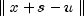• #### getPrimalInfeasibilityTolerance

`public double getPrimalInfeasibilityTolerance()`
Returns the primal infeasibility tolerance.
Returns:
a `double` scalar containing the primal infeasibility tolerance
• #### getPrintLevel

`public int getPrintLevel()`
Returns the print level. See `setPrintLevel`.
Returns:
an `int` scalar containing the print level
• #### getRelativeOptimalityTolerance

`public double getRelativeOptimalityTolerance()`
Returns the relative optimality tolerance.
Returns:
a `double` scalar containing the relative optimality tolerance
• #### getSmallestCPRatio

`public double getSmallestCPRatio()`
Returns the ratio of the smallest complementarity product to the average.
Returns:
a `double` scalar containing the ratio of the smallest complementarity product to the average
• #### getSolution

`public double[] getSolution()`
Returns the solution x of the linear programming problem.
Returns:
a `double` array containing the solution x of the linear programming problem
• #### getTerminationStatus

`public int getTerminationStatus()`
Returns the termination status for the problem.
Returns:
an `int` scalar containing the termination status for the problem  status Description 0 Optimal solution found. 1 The problem is primal infeasible (or dual unbounded). 2 The problem is primal unbounded (or dual infeasible). 3 Suboptimal solution found (accuracy problems). 4 Iterations limit `maxIterations` exceeded. 5 An error outside of the solution phase of the algorithm, e.g. a user input or a memory allocation error.
• #### getUpperBound

`public double[] getUpperBound()`
Returns the upper bound on the variables.
Returns:
a `double` array containing the upper bound on the variables
• #### getUpperLimit

`public double[] getUpperLimit()`
Returns the upper limit of the constraints that have both a lower and an upper bound.
Returns:
a `double` array containing the upper limit of the constraints that have both a lower and an upper bound. Returns `null` if the upper limit has not been set.
• #### getViolation

`public double getViolation()`
Returns the violation of the variable bounds.
Returns:
a `double` scalar containing the violation of the variable bounds,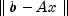• #### setConstant

`public void setConstant(double c0)`
Sets the value of the constant term in the objective function.
Parameters:
`c0` - a `double` scalar containing the value of the constant term in the objective function

Default: `c0 = 0`

• #### setConstraintType

`public void setConstraintType(int[] constraintType)`
Sets the types of general constraints in the matrix A.
Parameters:
`constraintType` - an `int` array of length m containing the types of general constraints in the matrix A. Let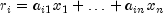. Then, the value of `constraintType[i]` signifies the following:  constraintType Constraint 0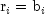1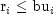2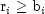3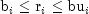4 Ignore this constraint

Note that `constraintType[i] = 3` should only be used for constraints `i` with both a finite lower and a finite upper bound. For one-sided constraints, use `constraintType[i] = 1` or `constraintType[i] = 2`. For free constraints, use `constraintType[i] = 4`.

Default: `constraintType[i] = 0`

• #### setDualInfeasibilityTolerance

`public void setDualInfeasibilityTolerance(double dualTolerance)`
Sets the dual infeasibility tolerance.
Parameters:
`dualTolerance` - a `double` scalar containing the dual infeasibility tolerance

Default: `dualTolerance = 1.0e-8`

• #### setLowerBound

`public void setLowerBound(double[] lowerBound)`
Sets the lower bound on the variables.
Parameters:
`lowerBound` - a `double` array of length n containing the lower boundon the variables. If there is no lower bound on a variable, then -1.0e30 should be set as the lower bound.

Default: `lowerBound[i] = 0`

• #### setMaxIterations

`public void setMaxIterations(int maxIterations)`
Sets the maximum number of iterations allowed for the primal-dual solver.
Parameters:
`maxIterations` - an `int` scalar containing the maximum number of iterations allowed for the primal-dual solver

Default: `maxIterations = 200`

• #### setPreordering

`public void setPreordering(int preorder)`
Sets the variant of the Minimum Degree Ordering (MDO) algorithm used in the preordering of the normal equations or augmented system matrix.
Parameters:
`preorder` - an `int` scalar containing the variant of the Minimum Degree Ordering (MDO) algorithm used in the preordering of the normal equations or augmented system matrix  preorder Method 0 A variant of the MDO algorithm using pivotal cliques. 1 A variant of George and Liu's Quotient Minimum Degree algorithm.

Default: `preorder = 0`

• #### setPresolve

`public void setPresolve(int presolve)`
Sets the presolve option.
Parameters:
`presolve` - an `int` containing the the presolve option to resolve the LP problem in order to reduce the problem size or to detect infeasibility or unboundedness of the problem. Depending on the number of presolve techniques used, different presolve levels can be chosen:  presolve Description 0 No presolving. 1 Eliminate singleton rows. 2 In addition to 1, eliminate redundant (and forcing) rows. 3 In addition to 1 and 2, eliminate dominated variables. 4 In addition to 1, 2, and 3, eliminate singleton columns. 5 In addition to 1, 2, 3, and 4, eliminate doubleton rows. 6 In addition to 1, 2, 3, 4, and 5, eliminate aggregate columns.

Default: `presolve = 0`

• #### setPrimalInfeasibilityTolerance

`public void setPrimalInfeasibilityTolerance(double primalTolerance)`
Sets the primal infeasibility tolerance.
Parameters:
`primalTolerance` - a `double` scalar containing the primal infeasibility tolerance

Default: `primalTolerance = 1.0e-8`

• #### setPrintLevel

`public void setPrintLevel(int printLevel)`
Sets the print level.
Parameters:
`printLevel` - an `int` containing the print level  printLevel Action 0 No printing. 1 Prints statistics on the LP problem and the solution process.

Default: `printLevel = 0`

• #### setRelativeOptimalityTolerance

`public void setRelativeOptimalityTolerance(double optimalityTolerance)`
Sets the relative optimality tolerance.
Parameters:
`optimalityTolerance` - a `double` scalar containing the relative optimality tolerance

Default: `optimalityTolerance = 1.0e-10`

• #### setUpperBound

`public void setUpperBound(double[] upperBound)`
Sets the upper bound on the variables.
Parameters:
`upperBound` - a `double` array of length n containing the upper boundon the variables. If there is no upper bound on a variable, then 1.0e30 should be set as the upper bound.

Default: None of the variables has an upper bound

• #### setUpperLimit

`public void setUpperLimit(double[] bu)`
Sets the upper limit of the constraints that have both a lower and an upper bound.
Parameters:
`bu` - a `double` array of length m containing the upper limitof the constraints that have both a lower and an upper bound. If such a constraint exists, then method `setConstraintType` must be used to define the type of the constraints. If `constraintType[i] != 3`, i.e. if constraint `i` is not two-sided, then the corresponding entry in `bu`, `bu[i]`, is ignored.

Default: None of the constraints has an upper limit

• #### solve

```public void solve()
throws SparseLP.DiagonalWeightMatrixException,
SparseLP.CholeskyFactorizationAccuracyException,
SparseLP.PrimalUnboundedException,
SparseLP.PrimalInfeasibleException,
SparseLP.DualInfeasibleException,
SparseLP.InitialSolutionInfeasibleException,
SparseLP.TooManyIterationsException,
SparseLP.ProblemUnboundedException,
SparseLP.ZeroColumnException,
SparseLP.ZeroRowException,
SparseLP.IncorrectlyEliminatedException,
SparseLP.IncorrectlyActiveException,
SparseLP.IllegalBoundsException```
Solves the sparse linear programming problem by an infeasible primal-dual interior-point method.
Throws:
`SparseLP.DiagonalWeightMatrixException` - is thrown if a diagonal element of the diagonal weight matrix is too small
`SparseLP.CholeskyFactorizationAccuracyException` - is thrown if the Cholesky factorization failed because of accuracy problems
`SparseLP.PrimalUnboundedException` - is thrown if the primal problem is unbounded
`SparseLP.PrimalInfeasibleException` - is thrown if the primal problem is infeasible
`SparseLP.DualInfeasibleException` - is thrown if the dual problem is infeasible
`SparseLP.InitialSolutionInfeasibleException` - is thrown if the initial solution for the one-row linear program is infeasible
`SparseLP.TooManyIterationsException` - is thrown if the maximum number of iterations has been exceeded
`SparseLP.ProblemUnboundedException` - is thrown if the problem is unbounded
`SparseLP.ZeroColumnException` - is thrown if a column of the constraint matrix has no entries
`SparseLP.ZeroRowException` - is thrown if a row of the constraint matrix has no entries
`SparseLP.IncorrectlyEliminatedException` - is thrown if one or more LP variables are falsely characterized by the internal presolver
`SparseLP.IncorrectlyActiveException` - is thrown if one or more LP variables are falsely characterized by the internal presolver
`SparseLP.IllegalBoundsException` - is thrown if the lower bound is greater than the upper bound
JMSLTM Numerical Library 7.2.0